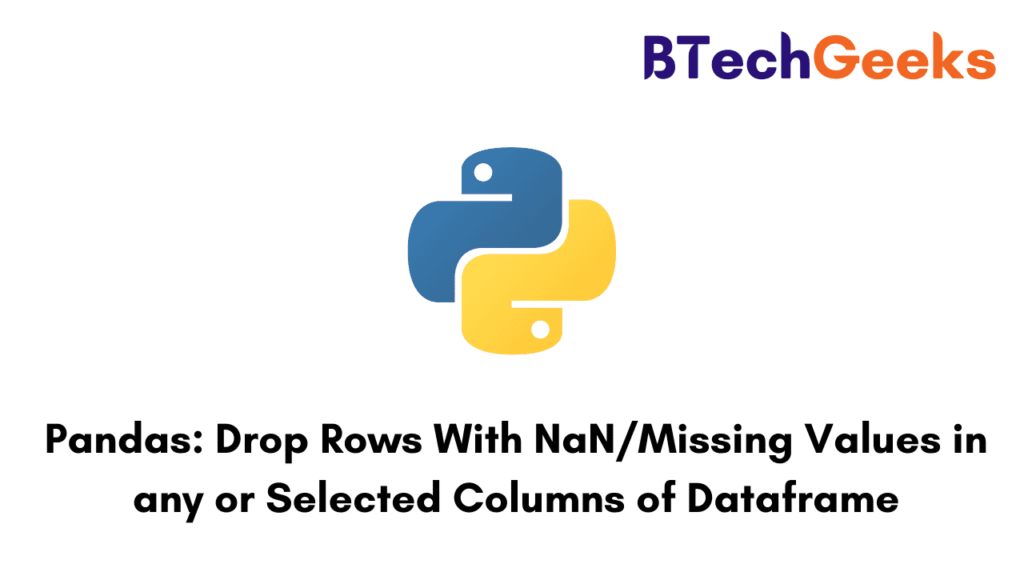# Pandas: Drop Rows With NaN/Missing Values in any or Selected Columns of Dataframe

Pandas provide several data structures and operations to manipulate data and time series. There might be instances in which some data can go missing and pandas use two values to denote the missing data namely None, NaN. You will come across what does None and Nan indicate. In this tutorial we will discuss the dropna() function, why is it necessary to remove rows which contain missing values or NaN, and different methods to drop rows with NaN or Missing values in any or selected column in the dataframe.

## dropna() function

The dropna() function is used to analyze and drop rows or columns having NaN or missing values in different ways.

syntax:  DataFrameName.dropna(axis, how, thresh, subset, inplace)

Parameters:

1) axis: If the axis is 0 rows with missing or NaN values will be dropped else if axis=1 columns with NaN or missing values will be dropped.

2) how: how to take a string as a parameter ‘any’ or ‘all’.  ‘any’ is used if any NaN value is present otherwise ‘all’ is used if all values are NaN.

3) thresh: It tells the minimum amount of NaN values that is to be dropped.

4) inplace: If inplace is true chance will be made in the existing dataset otherwise changes will be made in different datasets.

### The Necessity to remove NaN or Missing values

NaN stands for Not a Number. It is used to signify whether a particular cell contains any data or not. When we work on different datasets we found that there are some cells that may have NaN or missing values. If we work on that type of dataset then the chances are high that we do not get an accurate result. Hence while working on any dataset we check whether our datasets contain any missing values or not. If it contains NaN values we will remove it so as to get results with more accuracy.

## How to drop rows of Pandas DataFrame whose value in a certain column is NaN or a Missing Value?

There are different methods to drop rows of Pandas Dataframe whose value is missing or Nan. All 4 methods are explained with enough examples so that you can better understand the concept and apply the conceptual knowledge to other programs on your own.

### Method 1: Drop Rows with missing value / NaN in any column

In this method, we will see how to drop rows with missing or NaN values in any column. As we know in all our methods dropna() function is going to be used hence we have to play with parameters. By default value of the axis is 0 and how is ‘any’ hence dropna() function without any parameter will going to be used to drop rows with missing or NaN values in any column. Let see this with the help of an example.

import pandas as pd
import numpy as np
students = [('Raj', 24, 'Mumbai', 95) ,
('Rahul', 21, 'Delhi' , 97) ,
('Aadi', 22, np.NaN, 81) ,
('Abhay', np.NaN,'Rajasthan' , np.NaN) ,
('Ajjet', 21, 'Delhi' , 74)]
# Create a DataFrame object
df = pd.DataFrame(  students,
columns=['Name', 'Age', 'City', 'Marks'])
print("Original Dataframe\n")
print(df,'\n')
new_df=df.dropna()
print("New Dataframe\n")
print(new_df)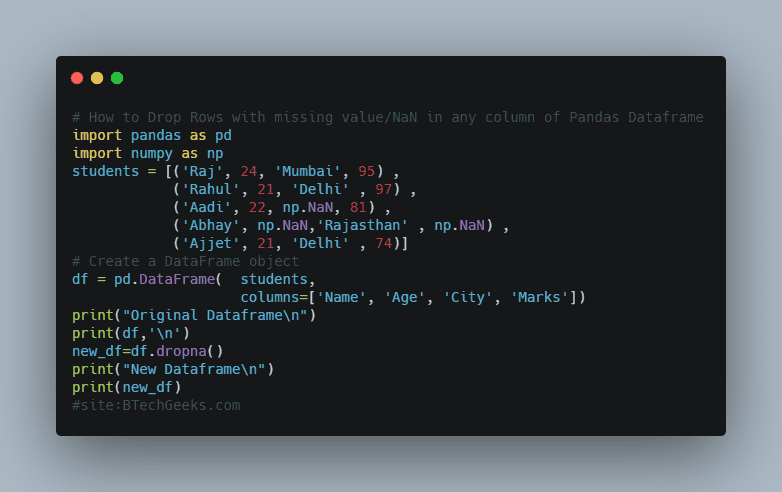Output

Original Dataframe

Name   Age       City  Marks
0    Raj  24.0     Mumbai   95.0
1  Rahul  21.0      Delhi   97.0
2   Aadi  22.0        NaN   81.0
3  Abhay   NaN  Rajasthan    NaN
4  Ajjet  21.0      Delhi   74.0

New Dataframe

Name   Age    City  Marks
0    Raj  24.0  Mumbai   95.0
1  Rahul  21.0   Delhi   97.0
4  Ajjet  21.0   Delhi   74.0

Here we see that we get only those rows that don’t have any NaN or missing value.

### Method 2: Drop Rows in dataframe which has all values as NaN

In this method, we have to drop only those rows in which all the values are NaN or missing. Hence we have to only pass how as an argument with value ‘all’ and all the parameters work with their default values. Let see this with an example.

import pandas as pd
import numpy as np
students = [('Raj', 24, 'Mumbai', 95) ,
('Rahul', 21, 'Delhi' , 97) ,
('Aadi', 22, np.NaN, 81) ,
('Abhay', np.NaN,'Rajasthan' , np.NaN) ,
('Ajjet', 21, 'Delhi' , 74),
(np.NaN,np.NaN,np.NaN,np.NaN),
('Aman',np.NaN,np.NaN,76)]
# Create a DataFrame object
df = pd.DataFrame(  students,
columns=['Name', 'Age', 'City', 'Marks'])
print("Original Dataframe\n")
print(df,'\n')
new_df=df.dropna(how='all')
print("New Dataframe\n")
print(new_df)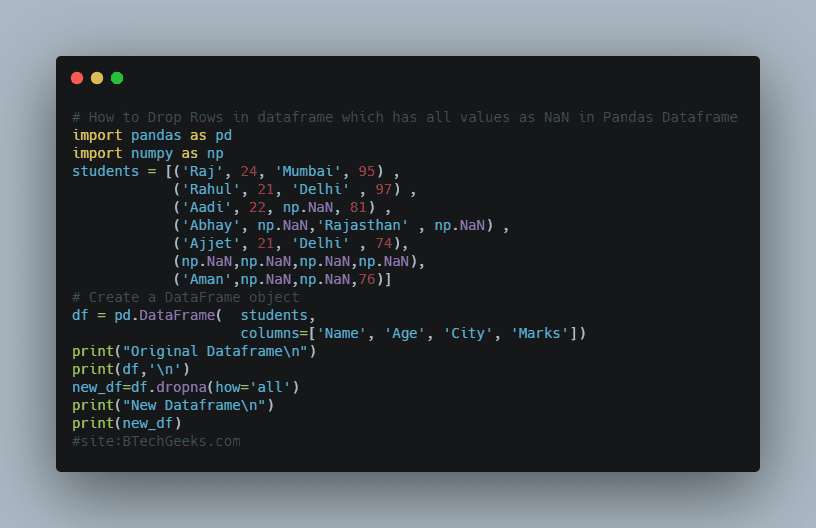Output

Original Dataframe

Name   Age       City  Marks
0    Raj  24.0     Mumbai   95.0
1  Rahul  21.0      Delhi   97.0
2   Aadi  22.0        NaN   81.0
3  Abhay   NaN  Rajasthan    NaN
4  Ajjet  21.0      Delhi   74.0
5    NaN   NaN        NaN    NaN
6   Aman   NaN        NaN   76.0

New Dataframe

Name   Age       City  Marks
0    Raj  24.0     Mumbai   95.0
1  Rahul  21.0      Delhi   97.0
2   Aadi  22.0        NaN   81.0
3  Abhay   NaN  Rajasthan    NaN
4  Ajjet  21.0      Delhi   74.0
6   Aman   NaN        NaN   76.0

Here we see that row 5 is dropped because it has all the values as NaN.

### Method 3: Drop Rows with any missing value in selected columns only

In this method, we see how to drop rows with any of the NaN values in the selected column only. Here also axis and how to take default value but we have to give a list of columns in the subset in which we want to perform our operation. Let see this with the help of an example.

import pandas as pd
import numpy as np
students = [('Raj', 24, 'Mumbai', 95) ,
('Rahul', 21, 'Delhi' , 97) ,
('Aadi', 22, np.NaN, 81) ,
('Abhay', np.NaN,'Rajasthan' , np.NaN) ,
('Ajjet', 21, 'Delhi' , 74),
(np.NaN,np.NaN,np.NaN,np.NaN),
('Aman',np.NaN,np.NaN,76)]
# Create a DataFrame object
df = pd.DataFrame(  students,
columns=['Name', 'Age', 'City', 'Marks'])
print("Original Dataframe\n")
print(df,'\n')
new_df=df.dropna(subset=['Name', 'Age'])
print("New Dataframe\n")
print(new_df)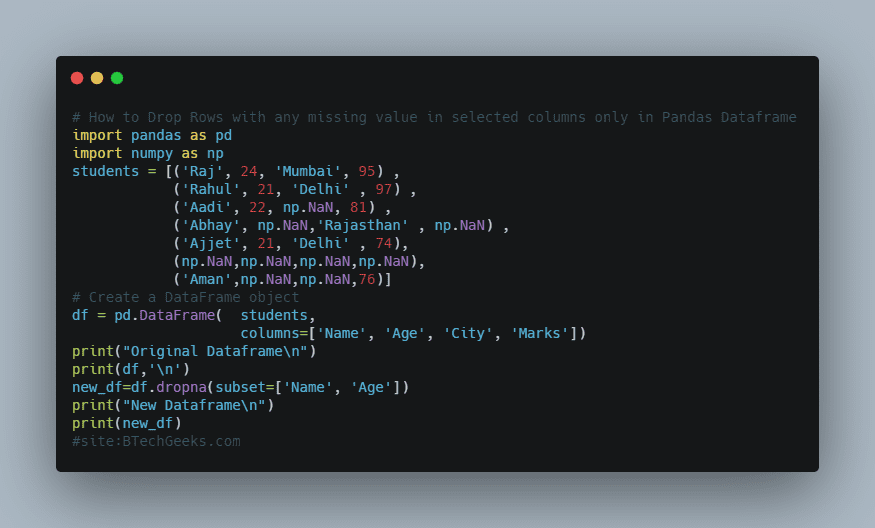Output

Original Dataframe

Name   Age       City  Marks
0    Raj  24.0     Mumbai   95.0
1  Rahul  21.0      Delhi   97.0
2   Aadi  22.0        NaN   81.0
3  Abhay   NaN  Rajasthan    NaN
4  Ajjet  21.0      Delhi   74.0
5    NaN   NaN        NaN    NaN
6   Aman   NaN        NaN   76.0

New Dataframe

Name   Age    City  Marks
0    Raj  24.0  Mumbai   95.0
1  Rahul  21.0   Delhi   97.0
2   Aadi  22.0     NaN   81.0
4  Ajjet  21.0   Delhi   74.0

Here we see in rows 3,5 and 6 columns ‘Name’ and ‘Age’ has NaN or missing values so these columns are dropped.

### Method 4: Drop Rows with missing values or NaN in all the selected columns

In this method we see how to drop rows that have all the values as NaN or missing values in a select column i.e if we select two columns ‘A’ and ‘B’ then both columns must have missing values. Here we have to pass a list of columns in the subset and ‘all’ in how. Let see this with the help of an example.

import pandas as pd
import numpy as np
students = [('Raj', 24, 'Mumbai', 95) ,
('Rahul', 21, 'Delhi' , 97) ,
('Aadi', 22, np.NaN, 81) ,
('Abhay', np.NaN,'Rajasthan' , np.NaN) ,
('Ajjet', 21, 'Delhi' , 74),
(np.NaN,np.NaN,np.NaN,np.NaN),
('Aman',np.NaN,np.NaN,76)]
# Create a DataFrame object
df = pd.DataFrame(  students,
columns=['Name', 'Age', 'City', 'Marks'])
print("Original Dataframe\n")
print(df,'\n')
new_df=df.dropna(how='all',subset=['Name', 'Age'])
print("New Dataframe\n")
print(new_df)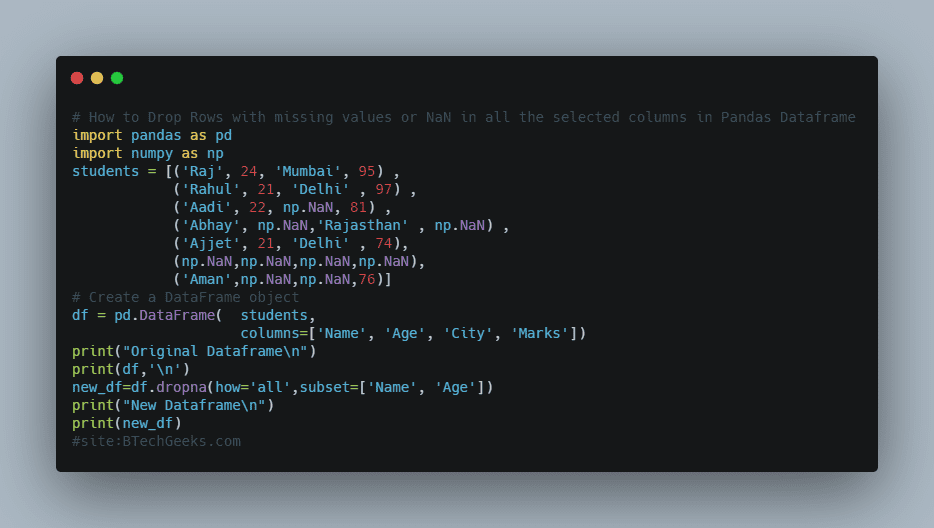Output

Original Dataframe

Name   Age       City  Marks
0    Raj  24.0     Mumbai   95.0
1  Rahul  21.0      Delhi   97.0
2   Aadi  22.0        NaN   81.0
3  Abhay   NaN  Rajasthan    NaN
4  Ajjet  21.0      Delhi   74.0
5    NaN   NaN        NaN    NaN
6   Aman   NaN        NaN   76.0

New Dataframe

Name   Age       City  Marks
0    Raj  24.0     Mumbai   95.0
1  Rahul  21.0      Delhi   97.0
2   Aadi  22.0        NaN   81.0
3  Abhay   NaN  Rajasthan    NaN
4  Ajjet  21.0      Delhi   74.0
6   Aman   NaN        NaN   76.0

Here we see that only row 7 has NaN value in both the columns hence it is dropped, while row 3 and row 6 have NaN value only in the age column hence it is not dropped.

So these are the methods to drop rows having all values as NaN or selected value as NaN.

Want to expert in the python programming language? Exploring Python Data Analysis using Pandas tutorial changes your knowledge from basic to advance level in python concepts.

Read more Articles on Python Data Analysis Using Pandas – Remove Contents from a Dataframe